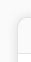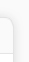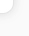About   People   Research   Publications   Software   Data   Blog   Join   Internal
 Stilianos Louca (2020), self-published. This book introduces advanced concepts of mathematical modeling in biology. The following topics are covered: Single- and multi-variate differential equation models (DEMs) Performing numerical simulations of DEMs Fitting DEMs to complex data via maximum-likelihood Linear DEM theory, matrix exponential, eigenvalues, normal modes, growth-transport models Forced linear DEMs, transfer function, impulse response functions Linear stability theory for DEMs Fourier analysis of models and of time series Epidemiological DEM models (e.g., SIR), basic reproduction ratio Vector Autoregression (VAR) models, stability Simulating and forecasting VAR models Fitting VAR models to data Stochastic processes, power spectral density The book targets senior undergraduate and graduate students in biology with a strong interest in mathematical methods, but could also be of interest to students in physics, mathematics and environmental sciences. Because this book aims to deliver generic modeling principles used across a wide range of situations in biology, readers from other disciplines will find that many of these principles are also applicable in their own field. This book may be freely used as reading material in related university courses or for self-teaching. Exercises are included throughout the book. References to literature covering additional material are also provided for the interested reader. All computational examples are provided in MATLAB, however the material is taught in a sufficiently general way that permits the use of alternative software such as python or R. Prerequisites: Recommended prerequisites for this book are familiarity with multivariate calculus (multivariate integrals, partial derivatives, basic differential equations), basic linear algebra (matrixes, eigenvectors, systems of linear equations), complex numbers, basic probability and statistics (common distributions, conditional probabilities), and basic experience with computer programming (any language). Readers lacking these prerequisites are strongly encouraged to fill these gaps beforehand, although Appendixes at the end of the book do provide quick introductions to some of these topics. Download: The latest edition of the book can be downloaded for free here.××××××